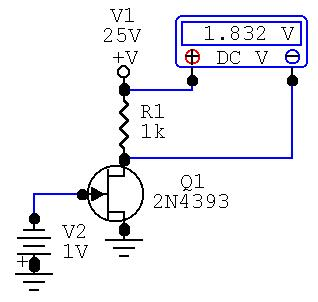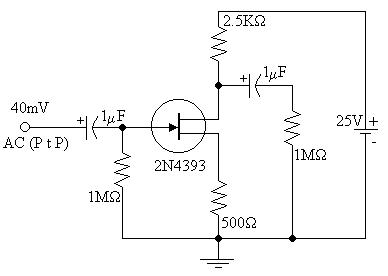EL - 250

Advanced Analog Circuits

Lab #3: Common Source JFET Amplifier

Andrew Buettner

March 5, 2003Objective

The objective of this lab is to determine the characteristics of a JFET, then to use that JFET in an audio amplifier to test it's operation by measuring the output and input voltages to calculate the theoretical and actual gain for a comparison.Equipment Used

No equipment necessary for this experiment. All data taken from computer simulations.Procedure

I: Part A

1) Assemble the following circuit:2) Run the simulation ensuring that the results are similar

3) Set VGS to .5V and record the results

II: Part B

1) Using the values obtained from part A to determine the VP for the theoretical JFET

2) Use the value calculated for VP to calculate IDSS

3) Using VGS and the calculated IDSS calculate ID

III: Part C

1) Assemble the following circuit:2) Determine ID, gm, the voltage gain, and the voltage output

IV: Part D

1) Load CSJFAMP.CKT in CircuitMaker©

2) Run the simulation and determine Vout

3) Compare the result with that of part CData

1) Table 1: Results from Part A

VGS

VRD

ID

1V

1.832V

1.832mA

.5V

8.362V

8.362mA

2) Table 2: Results from Part B

VGS

VP

IDSS

ID

1V

-1.44V

19.62mA

1.83mA

.5V

-1.44V

19.62mA

8.36mA

3) Table 3: Results from Part C

VOUT

ID

gm

AV

8.232V

1.968mA

90.6mS

205.8

4) Table 4: Comparison between Part C and Part D results

Part C:

Part D:

% error:

VOUT

8.232V

8.050V

2.211%

5) Diagram 1: Schematic, 1 Enlarged View6) Diagram 2: Schematic 2, Enlarged ViewConclusions

From this lab, it is shown how to use a JFET in an audio amplifier circuit and perform a small signal analysis. I would like to comment that the final voltage on the simulation may not be accurate as the voltmeter and oscilloscope were not giving accurate readings, either that or I was not reading them correctly as this is the first time I've ever used circuit maker. I was able to overcome all other problems as I feel that my calculations are fairly accurate (< 3% error from simulation.)Attachments

1) Original Lab Handout

2) Original Lab Data

3) Calculations